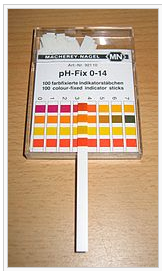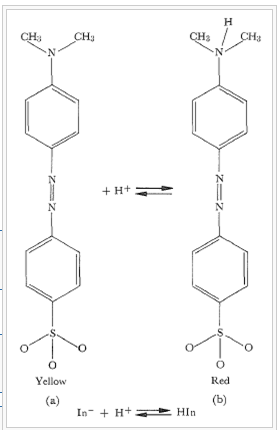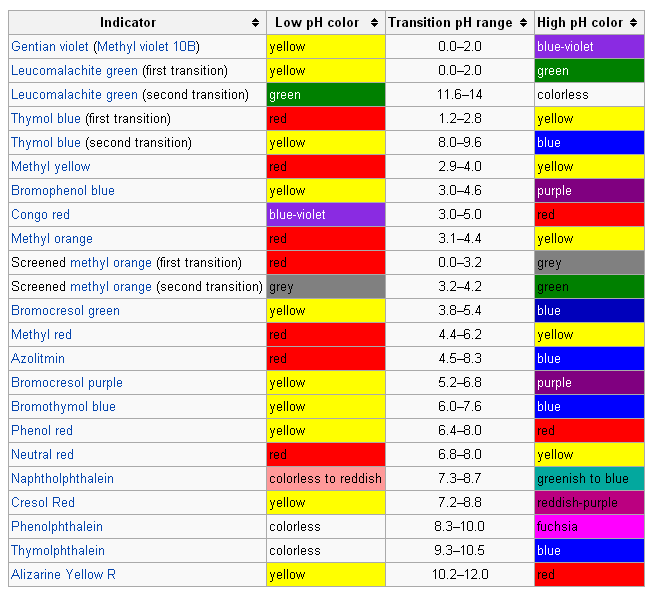## Acid-Base Indicators

#### Learning Objective

• Explain which, of a given series, would be the best acid-base indicator for a given titration.

#### Key Points

• In general, a molecule that changes color with the pH of the environment it is in can be used as an indicator.
• In the reaction $HIn\rightleftharpoons { H }^{ + } +{ In }^{ - }$ , adding acid shifts the indicator equilibrium to the left.
• In the reaction $HIn\rightleftharpoons { H }^{ + } +{ In }^{ - }$ , adding base shifts the indicator equilibrium to the right.
• For optimal accuracy, the color difference between the two colored species should be as clear as possible, and the narrower the pH range of the color change the better.

#### Terms

• pHThe negative of the logarithm to base 10 of the concentration of hydrogen ions, measured in moles per liter; a measure of acidity or alkalinity of a substance, which takes numerical values from 0 (maximum acidity) through 7 (neutral) to 14 (maximum alkalinity).
• indicatorA halochromic chemical compound that is added in small amounts to a solution so that the pH (acidity or basicity) of the solution can be determined visually.
• titrationThe determination of the concentration of some substance in a solution by slowly adding measured amounts of some other substance (normally using a burette) until a reaction is shown to be complete—for instance, by the color change of an indicator
• pH indicatorAn acid-base indicator.

There are many methods to determine the pH of a solution and to determine the point of equivalence when mixing acids and bases. These methods range from the use of litmus paper, indicator paper, specifically designed electrodes, and the use of colored molecules in solution. Other than the electrodes, all of the methods are visual and rely on some fundamental changes that occur in a molecule when the pH of its environment changes. In general, a molecule that changes color with the pH of the environment it is in can be used as an indicator.pH measuring stripspH can be determined to a reasonable level of accuracy by treating a strip with the solution to be tested and then observing the color sequence on the treated area.

In the equation:

$HIn \rightleftharpoons { H }^{ + }+ { In }^{ - }$

the weak acid HIn is shown in equilibrium with its ionized anion In. In this reaction, adding acid shifts the indicator equilibrium to the left. Conversely, adding a base shifts the indicator equilibrium to the right.

In the case of the indicator methyl orange, the HIn is colored red and the ionized In form is yellow.

In this example:

${ K }_{ a }\quad =\quad \frac { \left[ { H }^{ + } \right] \left[ { In }^{ - } \right] }{ \left[ HIn \right] }$

For methyl orange, Ka = 1.6 X 10-4 and pKa = 3.8. The neutral (red) and dissociated (yellow) forms of the indicator are present at equal concentrations when the pH = 3.8. The eye is sensitive to color changes over a range of concentration ratios of approximately 100 or over two pH units. Below pH 2.8, a solution containing methyl orange is red; above approximately 4.8, it is clearly yellow.Methyl orangeThe molecule methyl orange is commonly used as an indicator in acid-base equilibrium reactions. In base form, on the left in the figure, the color is yellow. Adding a proton yields the structure on the right, colored red. Note that this color change occurs over the pH range from approximately 3-4.

pH indicators are frequently employed in titrations in analytical chemistry and biology to determine the extent of a chemical reaction. Because of the subjective choice (determination) of color, pH indicators are susceptible to imprecise readings. For applications requiring precise measurement of pH, a pH meter is frequently used. Sometimes a blend of different indicators is used to achieve several smooth color changes over a wide range of pH values. These commercial indicators (e.g., universal indicator and Hydrion papers) are used when only rough knowledge of pH is necessary. Indicators usually exhibit intermediate colors at pH values inside a specific transition range. For example, phenol red exhibits an orange color between pH 6.8 and pH 8.4. The transition range may shift slightly depending on the concentration of the indicator in the solution and on the temperature at which it is used.Common acid-base indicatorsCommon indicators for pH indication or titration endpoints is given, with high, low, and transition pH colors.

When viewed on the pH scale itself, the color transitions as determined by their transition ranges becomes clearer and the context of the indicator sensitivity over ranges of pH is laid out more informatively.

Example:

In the titration of a weak acid with a strong base, which indicator would be the best choice?

A. Methyl Orange

B. Bromocresol Green

C. Phenolphtalein

The correct answer is C. In the titration of a weak acid with a strong base, the conjugate base of the weak acid will make the pH at the equivalence point greater than 7. Therefore, you would want an indicator to change in that pH range. Both methyl orange and bromocresol green change color in an acidic pH range, while phenolphtalein changes in a basic pH.